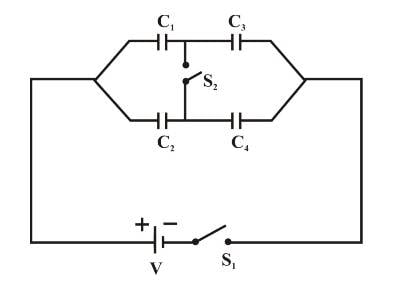# Charge on Capacitors

Falcons

## Homework Statement

I am asked to find the charge on each cap in this circuit when S1 is closed:I am given that C1=1μF, C2=2μF, C3=3μF, and C4=4μF. V=12V

## Homework Equations

Caps in series have an equivalent capacitance of Ceq=C1C2/(C1+C2), and caps in parallel have an equivalent capacitance of Ceq=C1+C2. The capacitance equals the voltage across the cap times the charge "held" in the cap. Parallel capacitors have the same voltage, whereas caps in series have the same charge.

## The Attempt at a Solution

I found the equivalent capacitance of the circuit to be 2.083μF, and using that and C=QV, found that the total charge on the circuit is 25μC. I worked backwards from there, knowing that caps in parallel have the same V, and found the charge on each equivalent cap for the series to be 9μC for C13 and C24. This is where I get lost. The book has the answer to be 9μC on both C1 and C3 and 16μC for C2 and C4. Assuming that I found the correct charge on the circuit, 25μC, this violates the law of charge conservation, doesn't it? Any help is appreciated, thanks.

Last edited:

Mentor
If S2 is not closed then there are no caps in parallel. So I wonder what it was you were calculating?

Falcons
Aren't C13 and C24 in parallel?

Edit: There is a second part to the problem that involved finding the charges on the caps when S2 is engaged. I also got half the charge the book has.

Last edited:
Mentor
Aren't C13 and C24 in parallel?

Ah. Yes, sorry, if you combine C1 and C3 into C13, and similarly combine C2 and C4 into C24, then those equivalent capacitors are indeed in parallel.

But it seems to be taking you a rather roundabout trip to the result to "lose" the components you're looking for the charges on, only to have to recover them again later on in the process.

Consider that C1 and C3 form a capacitive voltage divider, as do C2 and C4. You can look at each capacitive voltage divider separately to find the charges on their capacitors.

Falcons
We haven't covered capacitive voltage dividers yet in our class, so you lost me, haha. Is it something worth looking up and learning on my own or should I wait for the class to cover it?

Mentor
We haven't covered capacitive voltage dividers yet in our class, so you lost me, haha. Is it something worth looking up and learning on my own or should I wait for the class to cover it?

Definitely worth knowing. It'll get you to a result quickly without all that futzing around with pushing charges from here to there.

Essentially what happens with capacitors in series is that, if charge is "pushed" onto the first plate of the first capacitor, it pushes an equal charge off of its second plate and onto the first plate of the second capacitor, which in turn displaces an equal charge from the second plate of the second capacitor (whew!). Boiling it all down, series connected capacitors will all end up with the same charge on them.

You know how parallel capacitors all have the same voltage? Well serial capacitors all have the same charge.

If you do the algebra, when the smoke clears the voltage divides inversely as the capacitance. So, for two capacitors C1 and C2 in series, with voltage V across the series pair, then V1 = V*C2/(C1 + C2) and V2 = V*C1/(C1 + C2).

Last edited:
Falcons
V1 = V*C2/(C1 + C2) and V2 = V*C1/(C1 + C2).

I knew the whole bit about serial caps having the same charge, and I've seen this equation before but didn't know where it came from nor how to derive it. Thanks for the tip! I'll see what I can come up with using what you gave me here.

Falcons
Sorry for the double post, but I realized that I was being stupid. I guess it happens to all of us, no?
In a series of caps, Q is the same on all the caps in the series and is equal to the Q on the equivalent cap. Thanks for the help and the useful equations!